# Direction fields¶

In :
t, w, x, y, n = var('t w x y n')
palette = [(215/255, 0/255, 132/255), (255/255, 1/255, 73/255), (255/255, 121/255, 1/255), (255/255, 210/255, 0/255)]
cool_palette = [(0/255, 150/255, 173/255), (0/255, 200/255, 146/255)]


## Filling a water tank¶

### Scenario¶

You're pumping water into the bottom of a cylindrical tank. You want to know $y(t)$, the water level after $t$ seconds of pumping. The tank starts out empty. The pump pushes water into the tank with a constant pressure. As the water level rises, the pressure working against the pump grows proportionally.

### Example model¶

Let's suppose the flow rate is proportional to the pressure difference between the tank and the pump. (This assumption is based on the "Hagen–Poiseuille law," which describes flow through a long, narrow pipe.) If the pump pressure is $100$ (in kilopascals), the tank pressure is $10y$ (in kilopascals per meter), and the constant of proportionality is $\tfrac{1}{500}$ (in meters per minute per kilopascal), the flow rate is related to the amount of water in the tank by the equation

$$y' = \tfrac{1}{500}(100 - 10y).$$

In :
water_direction = plot_slope_field((1/500) * (100 - 10*y), (t, 0, 150), (y, 0, 12))
water_solutions = [plot(exp(-t/50) * 4*k + 10 * (1 - exp(-t/50)), (t, 0, 150), thickness = 3, rgbcolor = palette[k]) for k in range(4)]
water_labels = ["$t$ : seconds", "$y(t)$ : meters"]
show(water_direction, axes_labels = water_labels)
show(water_direction + water_solutions, axes_labels = water_labels)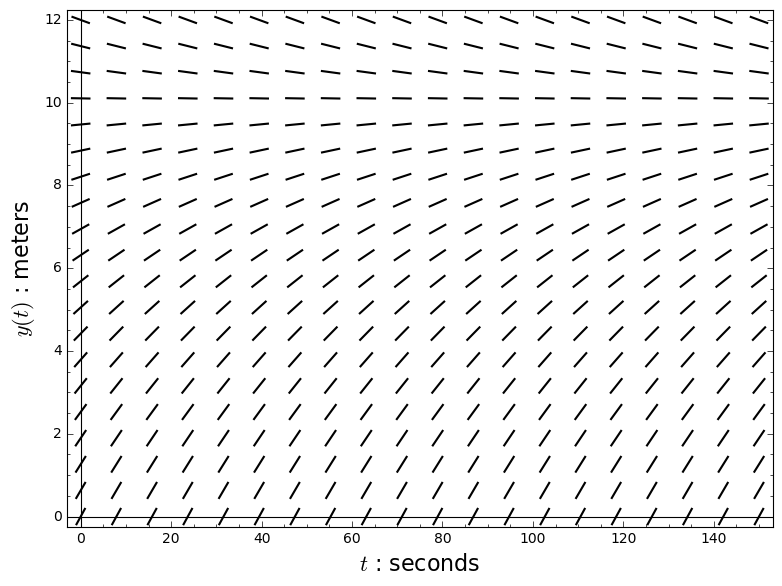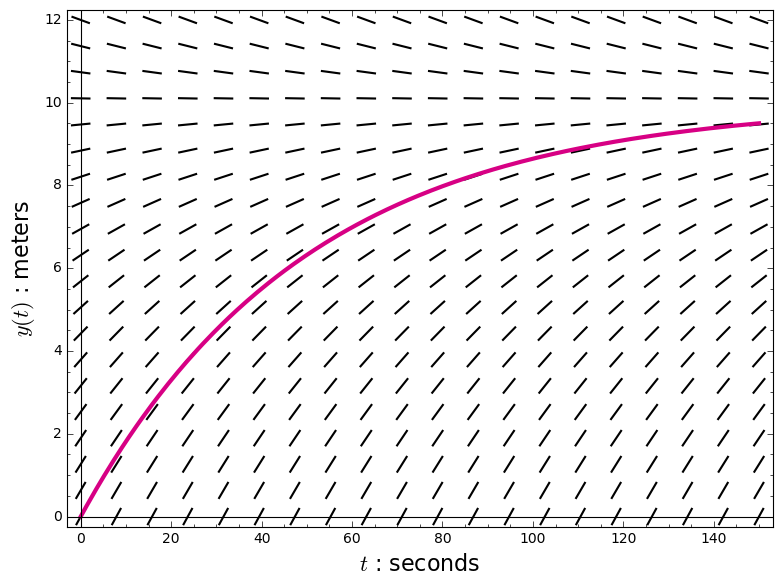### Other initial water levels¶

What if the tank starts out with some water in it?

In :
show(water_direction + sum(water_solutions), axes_labels = water_labels)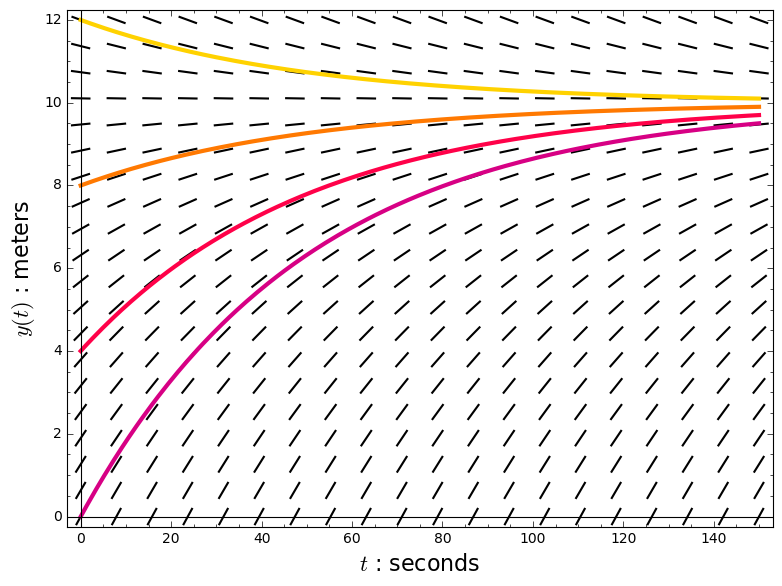## Wearing out truck tires¶

### Scenario¶

You order a batch of new tires for a very large fleet of trucks. You want to know $n(t)$, the number of tires that are still intact after $t$ distance units of driving.

### Example model¶

$$n' = -5t^4 n$$

In :
tires_direction = plot_slope_field(-5*t^4 * n, (t, 0, 2), (n, 0, 4000))
tires_solutions = [plot(1000*(4-k) * exp(-t^5), (t, 0, 2), thickness = 3, rgbcolor = palette[k]) for k in range(4)]
tires_labels = ["$t$ : distance units", "$n(t)$ : tires"]
show(tires_direction, axes_labels = tires_labels)
show(tires_direction + tires_solutions, axes_labels = tires_labels)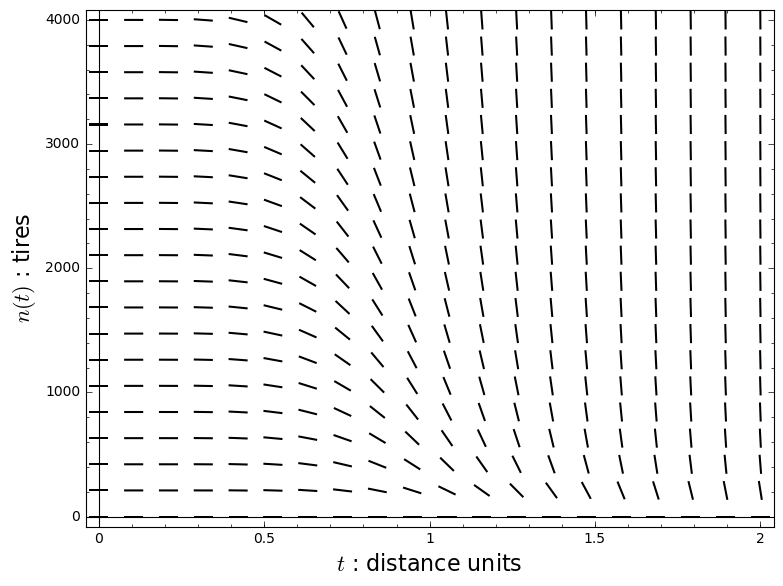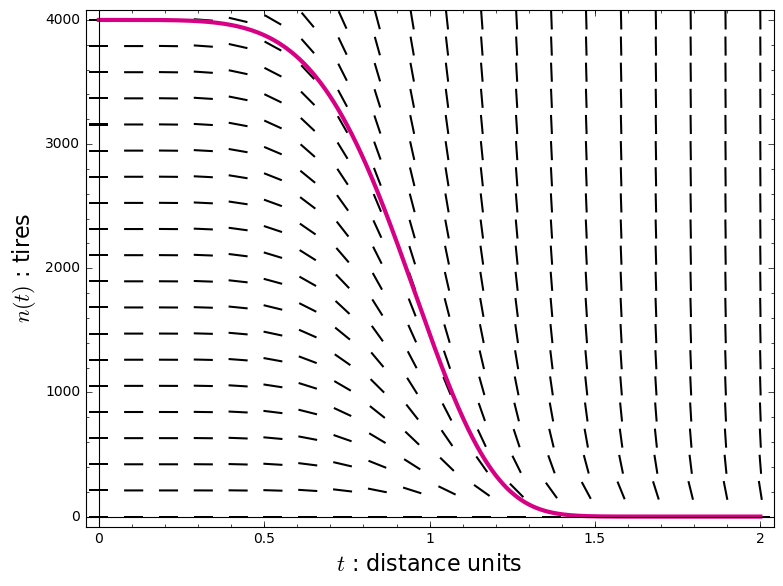### Other initial numbers of tires¶

What if you start out with fewer tires?

In :
show(tires_direction + sum(tires_solutions), axes_labels = tires_labels)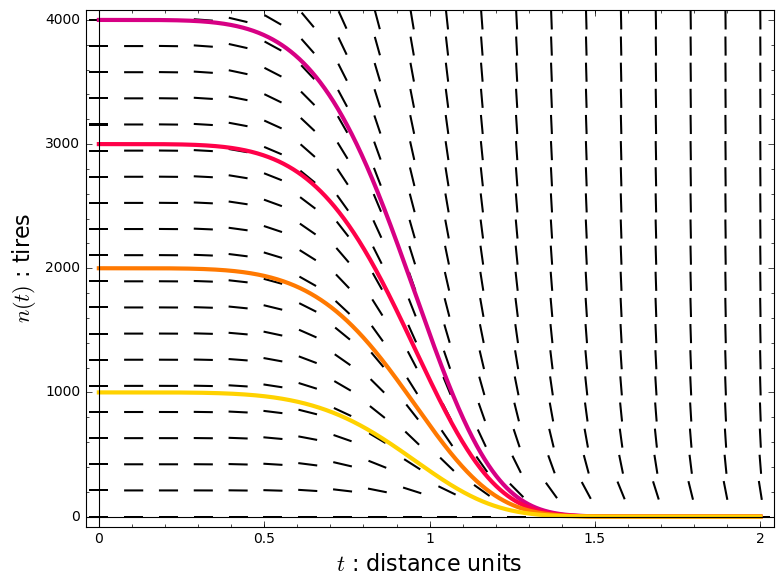## Swinging a pendulum¶

### Scenario¶

You attach a heavy weight to the ceiling using a light string. You pull the weight 60° back from vertical, keeping the string taut, and let it go. You want to know $\theta(t)$, the angle of the string after $t$ seconds of swinging.

### Example model¶

$$\theta'' = -\sin \theta$$

In :
energies = [1 - cos(k*pi/12) for k in reversed(range(1,5))]
pendulum_solutions = [plot(asin(sqrt(en/2) * jacobi_sn(t, en/2)), (t, elliptic_kc(en/2), 9*elliptic_kc(en/2)), thickness = 3, rgbcolor = color) for (en, color) in zip(energies, palette)]
pendulum_labels = ["$t$ : seconds", "$\\theta(t)$ : radians"]
show(pendulum_solutions, axes_labels = pendulum_labels)